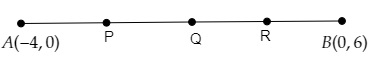# Find the coordinates of the points which divide the line segment joining the points $(-4, 0)$ and $(0, 6)$ in four equal parts.

Given:

The line segment joining the points $(-4, 0)$ and $(0, 6)$ is divided into four equal parts.

To do:

We have to find the coordinates of the points which divide the line segment joining the points $(-4, 0)$ and $(0, 6)$ in four equal parts.

Solution:

Let $AB$ be a line segment whose ends points are $A (-4, 0)$ and $B (0, 6)$.Let $P, Q, R$ be the points which divide $AB$ in four equal parts.

This implies,

$A P=P Q=Q R=R B$

$Q$ is the mid-point of $\mathrm{AB}$ and $\mathrm{P}$ and $\mathrm{R}$ are the mid points of $A Q$ and $Q B$ respectively.

Using mid-point formula, we get,

The coordinates of $\mathrm{Q}= \left(\frac{-4+\dot{0}}{2}, \frac{0+6}{2}\right)$

$=\left(\frac{-4}{2}, \frac{6}{2}\right)$

$=(-2,3)$

The coordinates of $\mathrm{P}= \left(\frac{-4-2}{2}, \frac{0+3}{2}\right)$

$=\left(\frac{-6}{2}, \frac{3}{2}\right)$

$=\left(-3, \frac{3}{2}\right)$

$=(-3,1.5)$

The coordinates of $\mathrm{R}= \left(\frac{-2+0}{2}, \frac{3+6}{2}\right)$

$=\left(\frac{-2}{2}, \frac{9}{2}\right)$

$=(-1,4.5)$

Therefore, the coordinates of the required points are $\left(-3, 1.5\right), (-2,3)$ and $(-1,4.5)$.

Updated on: 10-Oct-2022

39 Views